﻿ Geometric Figures, Circle Icon. Elements Of Geometric Figures Illustration Icon. Signs And Symbols Can Be Used For Web, Logo, Stock Vector - Illustration of graphic, perspective: 145501025

# Geometric figures, circle icon. Elements of geometric figures illustration icon. Signs and symbols can be used for web, logo, .

## Geometric figures, circle icon. Elements of geometric figures illustration icon. Signs and symbols can be used for web, logo, mobile app, UI, UX on white background

More similar stock illustrations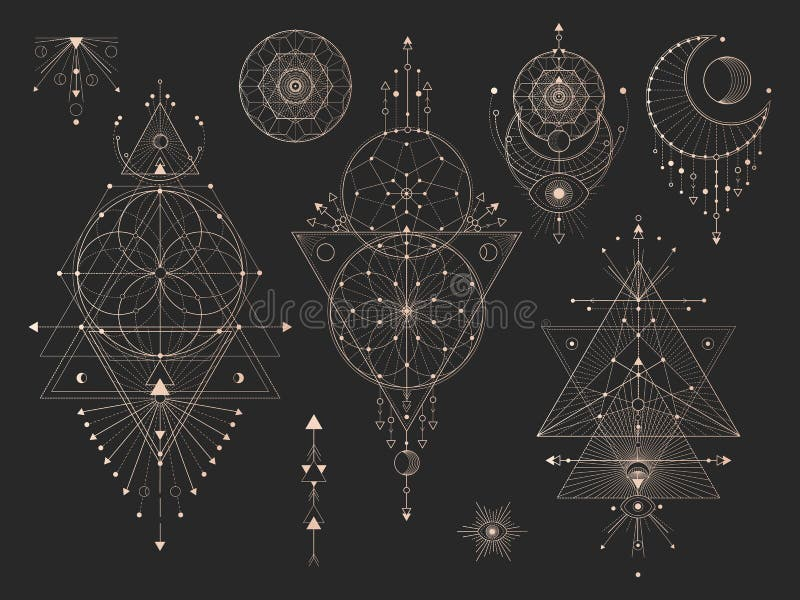Vector set of Sacred geometric symbols with moon, eye, arrows, dreamcatcher and figures on black background. Gold abstract mystic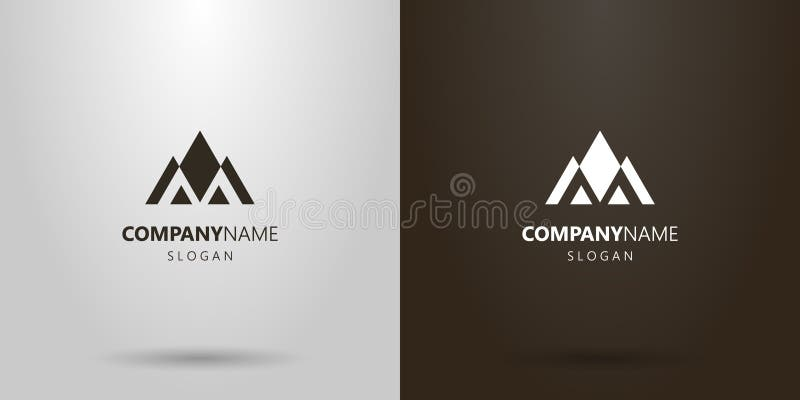Simple vector geometric logo of a mountain landscape of triangular figures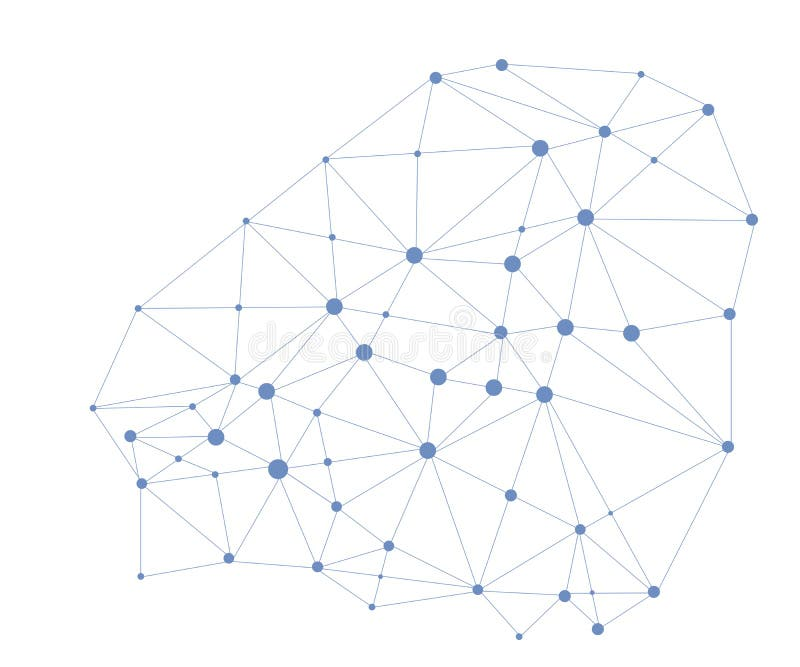Linked Geometric Wire frame Figures lines connected with Dots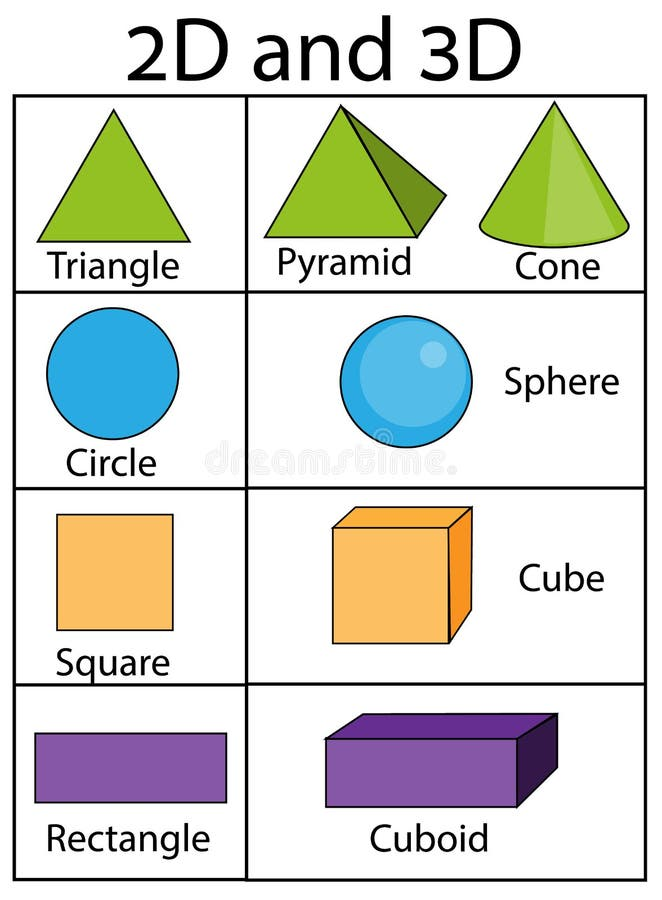2D and 3D geometric shapes and figures. Educational infographics for kids. Children visual aids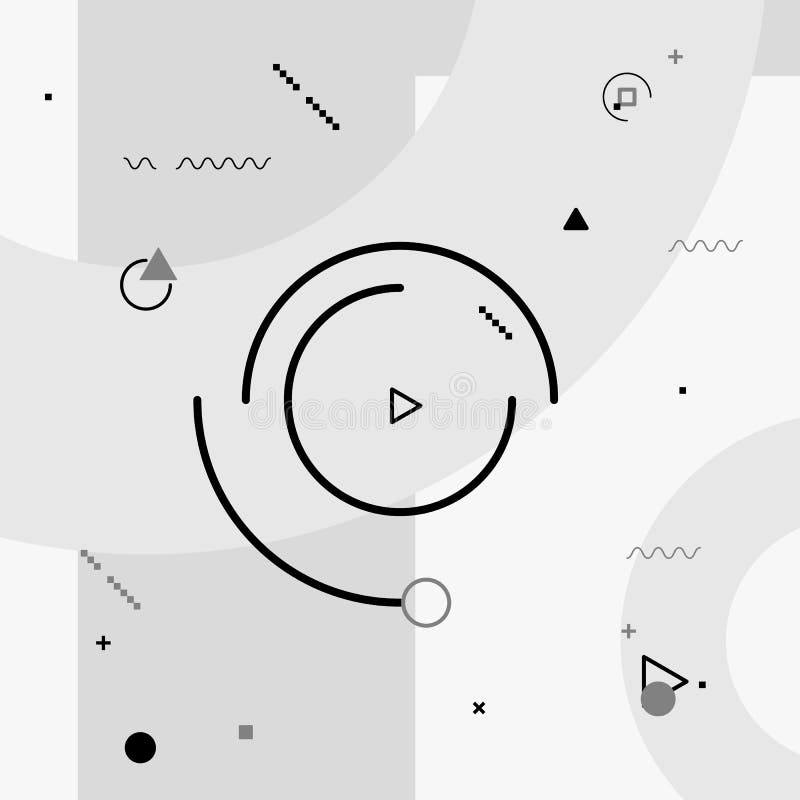Motion graphics elements. Black and white composition. Vector illustration background. Geometric figures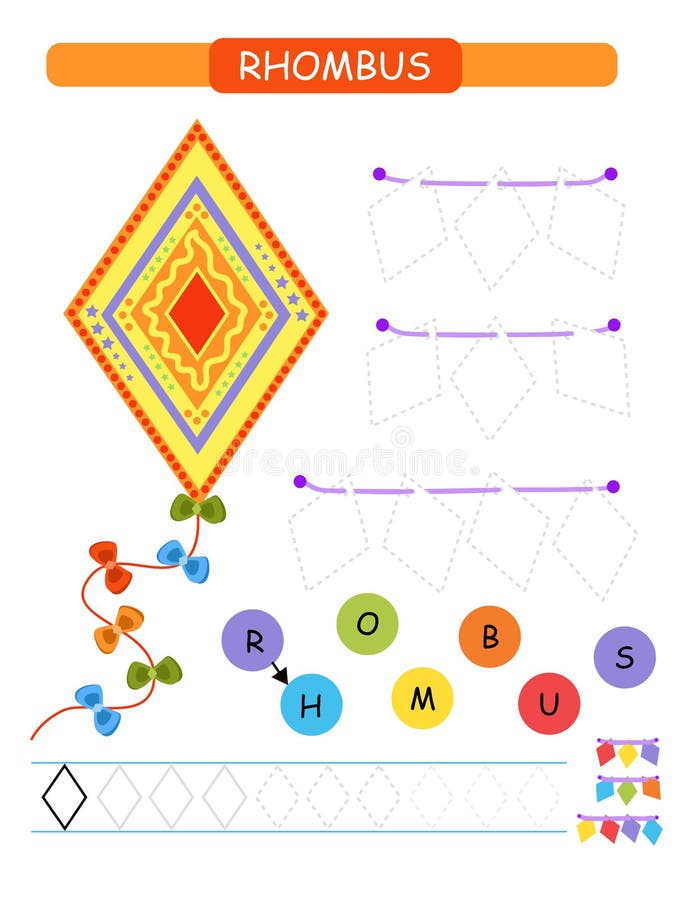Learn shapes and geometric figures for preschool and kindergarten printable worksheet. Cartoon vector illustration -rhombus.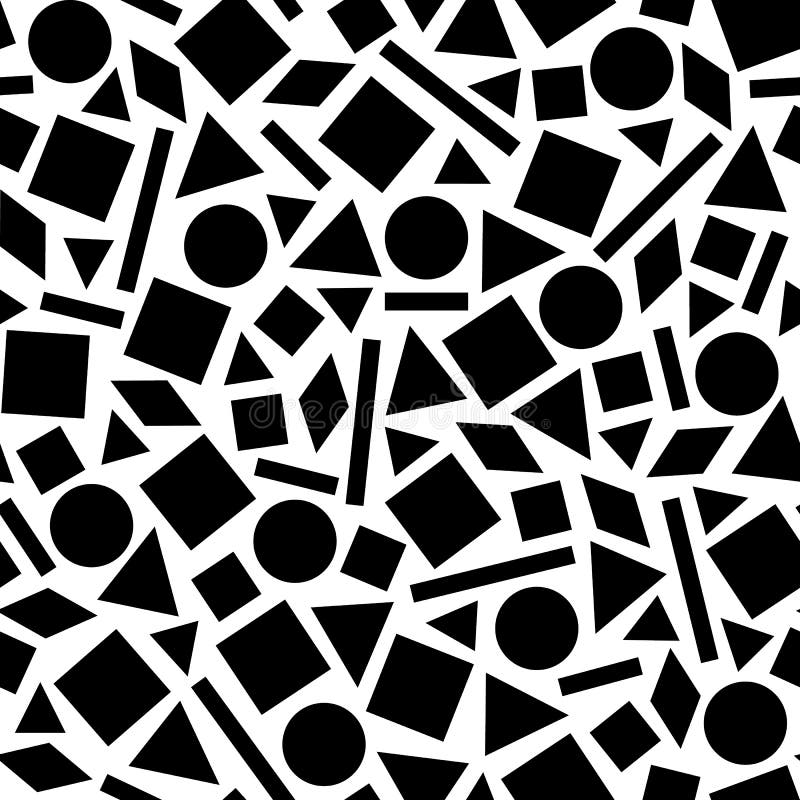Geometric pattern of the figures.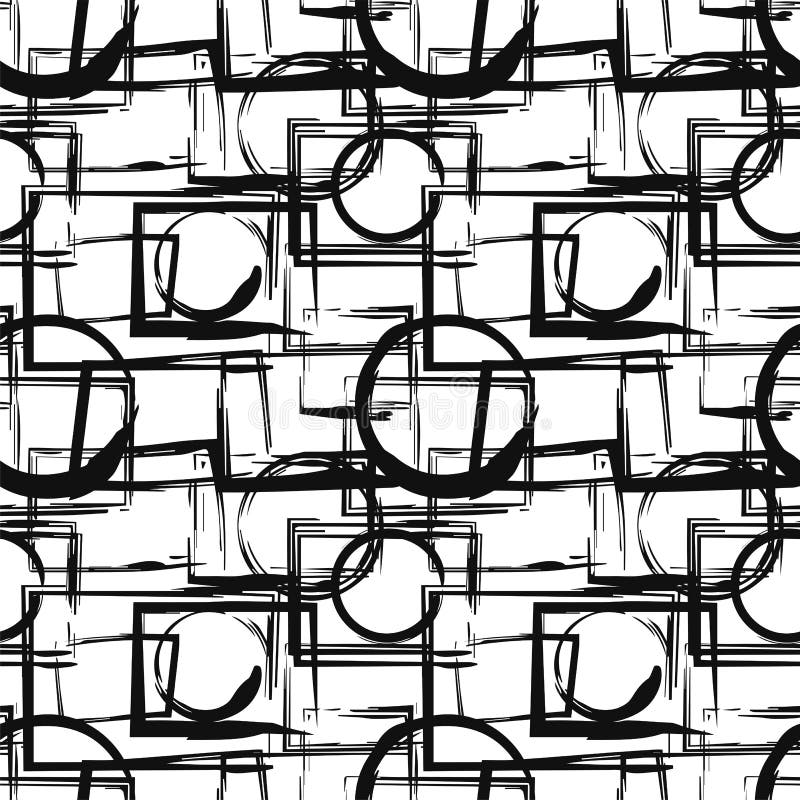Seamless pattern with abstract black geometric figures in grunge style. Vector design elements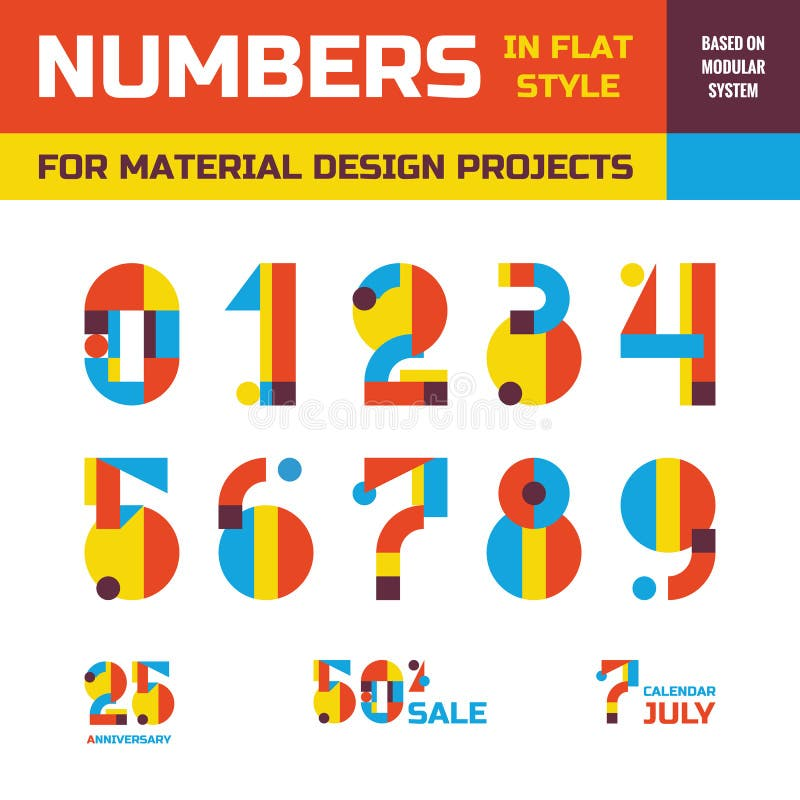Abstract vector numbers in flat style design for material design creative projects. Geometric numbers symbols. Decorative figures.
More stock photos from Fidan Babayeva's portfolio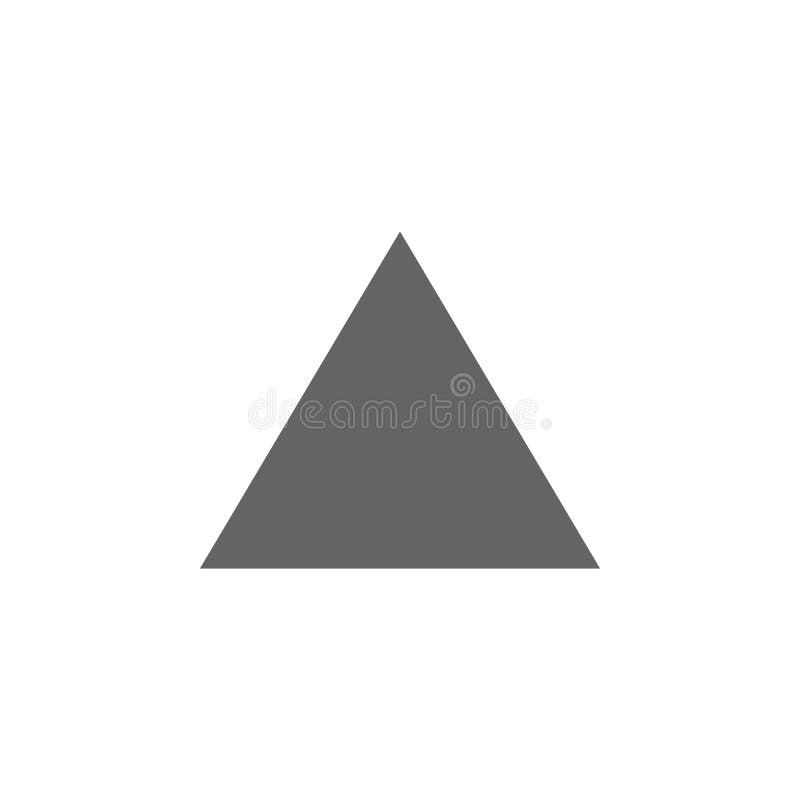Geometric figures, triangle icon. Elements of geometric figures illustration icon. Signs and symbols can be used for web, logo,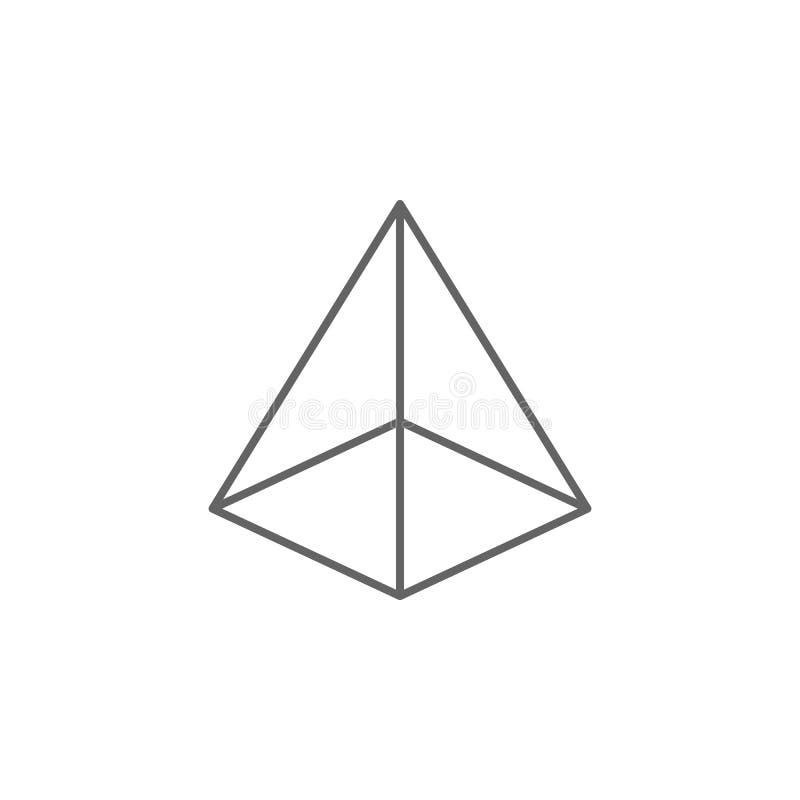Geometric figures, square based pyramid outline icon. Elements of geometric figures illustration icon. Signs and symbols can be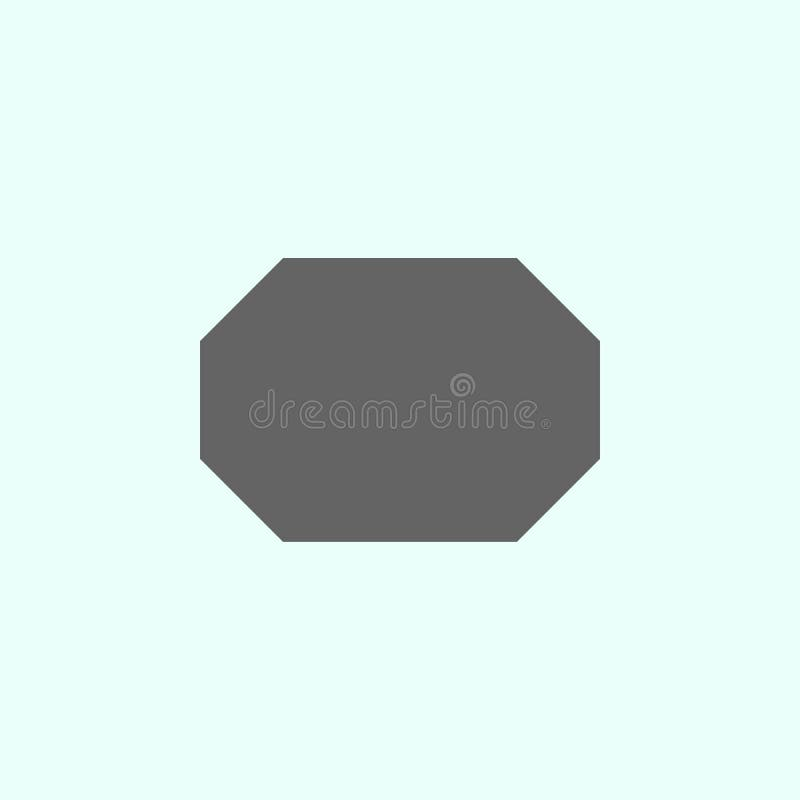Geometric figures, octagon icon. Elements of geometric figures illustration icon. Signs and symbols can be used for web, logo,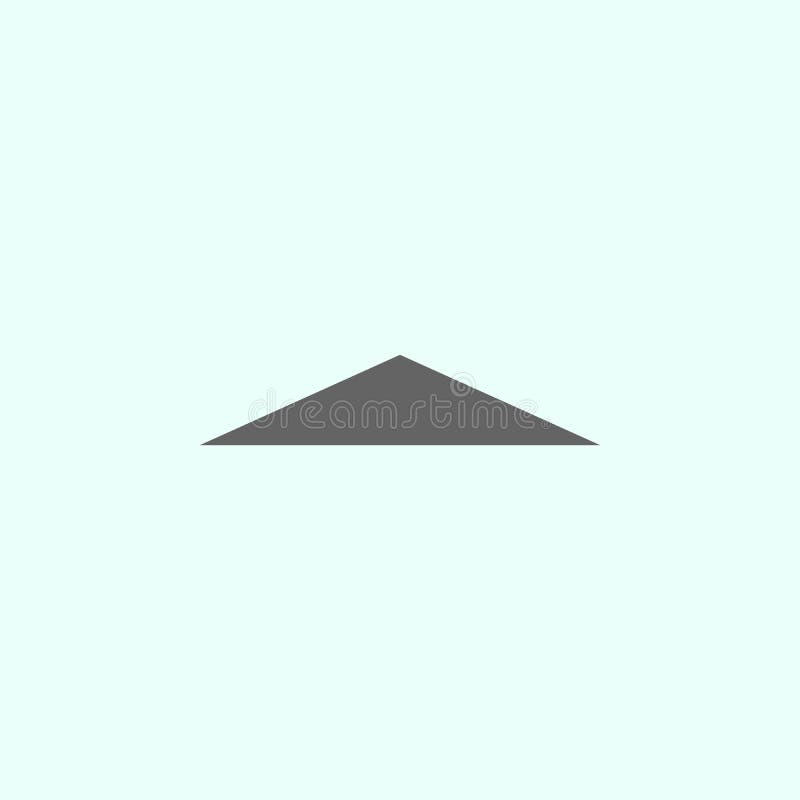Geometric figures, isosceles triangle icon. Elements of geometric figures illustration icon. Signs and symbols can be used for web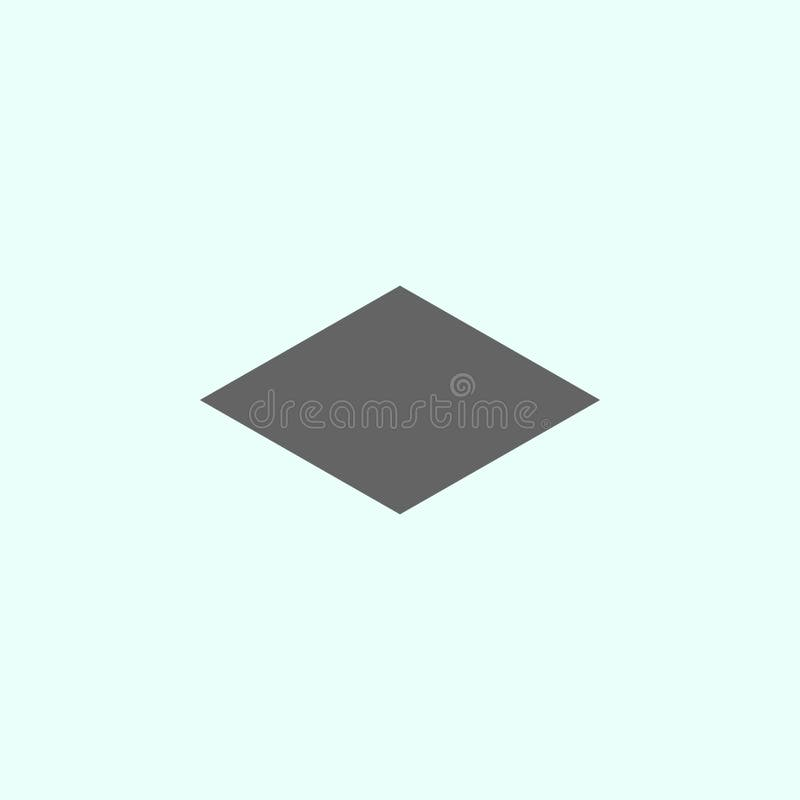Geometric figures, rhombus icon. Elements of geometric figures illustration icon. Signs and symbols can be used for web, logo,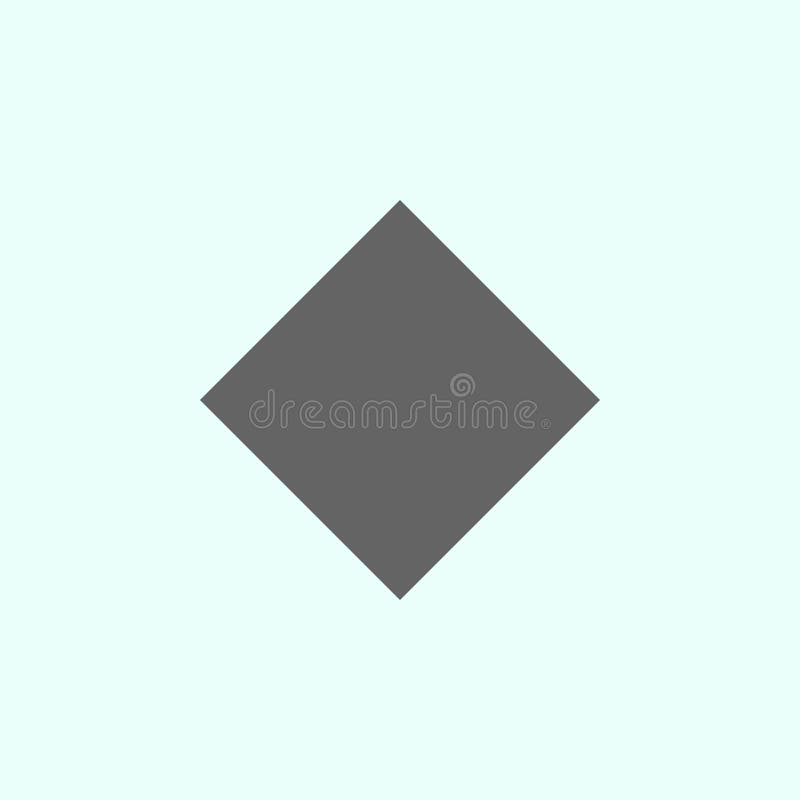Geometric figures, rhombus icon. Elements of geometric figures illustration icon. Signs and symbols can be used for web, logo,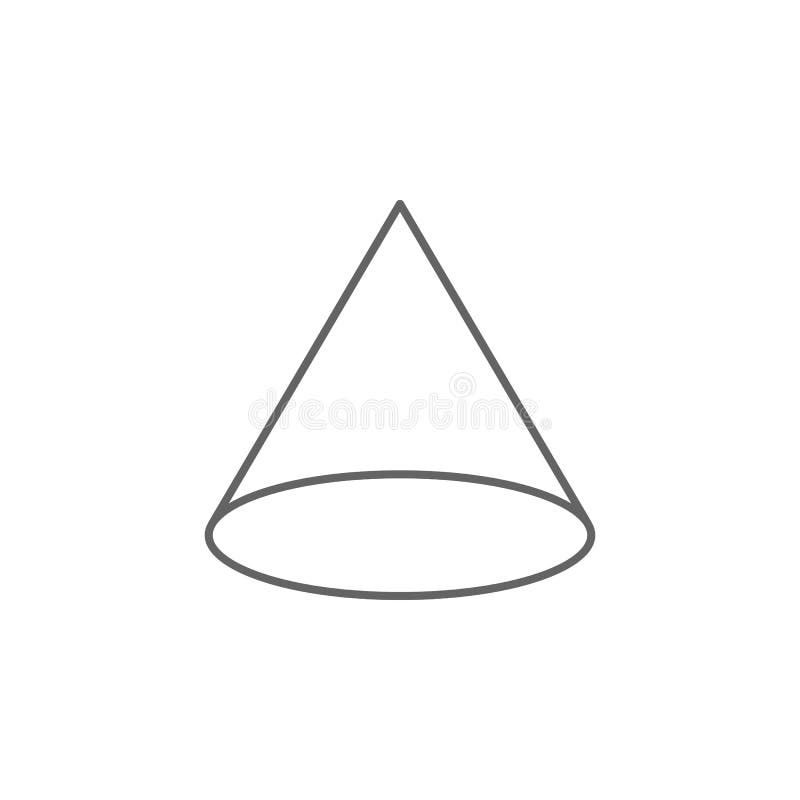Geometric figures, cone outline icon. Elements of geometric figures illustration icon. Signs and symbols can be used for web, logo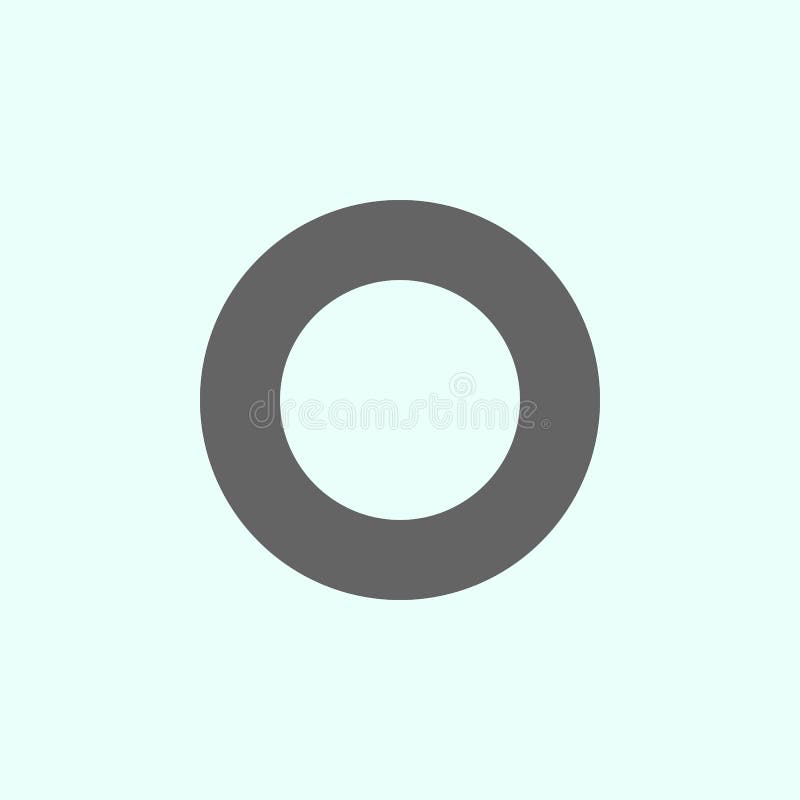Geometric figures, ring icon. Elements of geometric figures illustration icon. Signs and symbols can be used for web, logo, mobile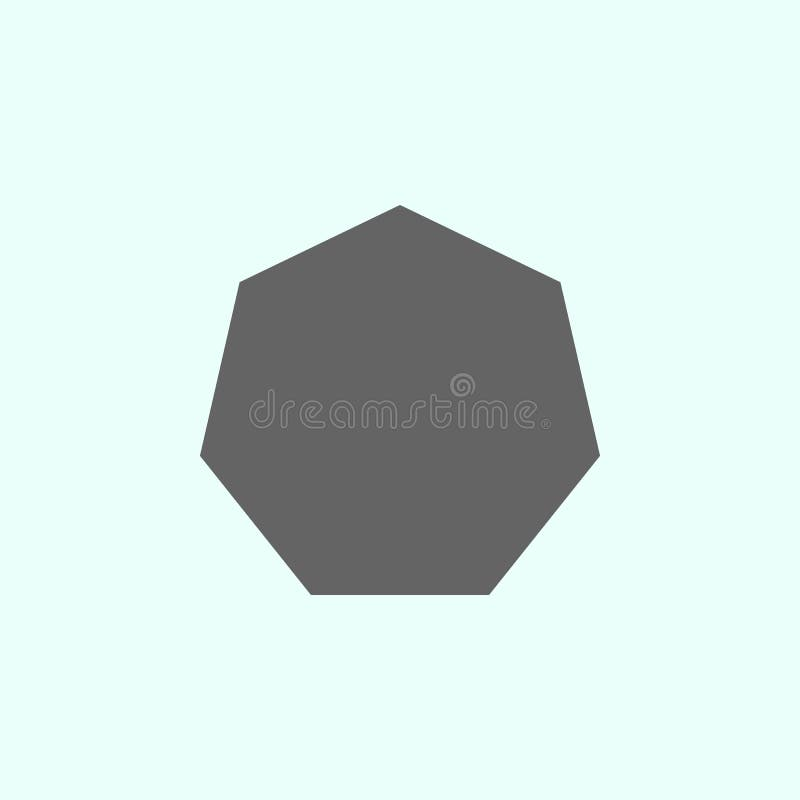Geometric figures, heptagon icon. Elements of geometric figures illustration icon. Signs and symbols can be used for web, logo,
Related categories